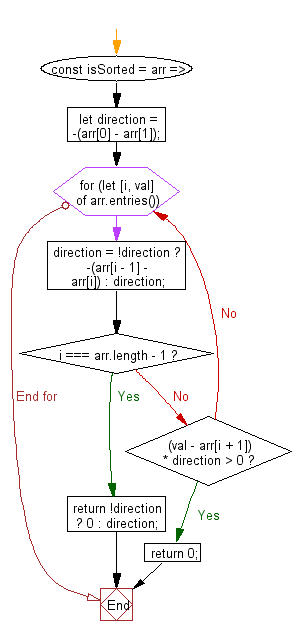# JavaScript: Return 1 if the array is sorted in ascending order, -1 if it is sorted in descending order or 0 if it is not sorted

## JavaScript fundamental (ES6 Syntax): Exercise-188 with Solution

Write a JavaScript program that returns 1 if the array is sorted in ascending order. It returns -1 if it is sorted in descending order or 0 if it is not sorted.

• Calculate the ordering direction for the first pair of adjacent array elements.
• Return 0 if the given array is empty, only has one element or the direction changes for any pair of adjacent array elements.
• Use Math.sign() to covert the final value of direction to -1 (descending order) or 1 (ascending order).

Sample Solution:

JavaScript Code:

``````//#Source https://bit.ly/2neWfJ2
const isSorted = arr => {
let direction = -(arr - arr);
for (let [i, val] of arr.entries()) {
direction = !direction ? -(arr[i - 1] - arr[i]) : direction;
if (i === arr.length - 1) return !direction ? 0 : direction;
else if ((val - arr[i + 1]) * direction > 0) return 0;
}
};
console.log(isSorted([0, 1, 2, 2]));
console.log(isSorted([4, 3, 2]));
console.log(isSorted([4, 3, 5]));
```
```

Sample Output:

```1
-1
0
```

Pictorial Presentation:Flowchart:Live Demo:

See the Pen javascript-basic-exercise-188-1 by w3resource (@w3resource) on CodePen.

Improve this sample solution and post your code through Disqus

What is the difficulty level of this exercise?

Test your Programming skills with w3resource's quiz.

﻿